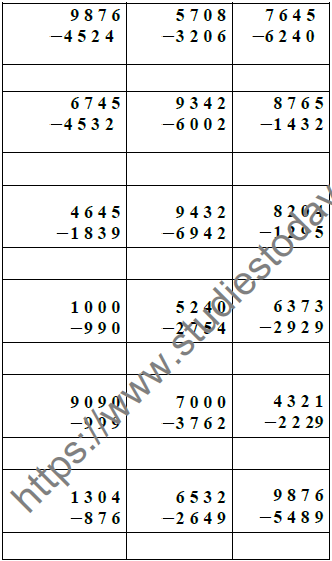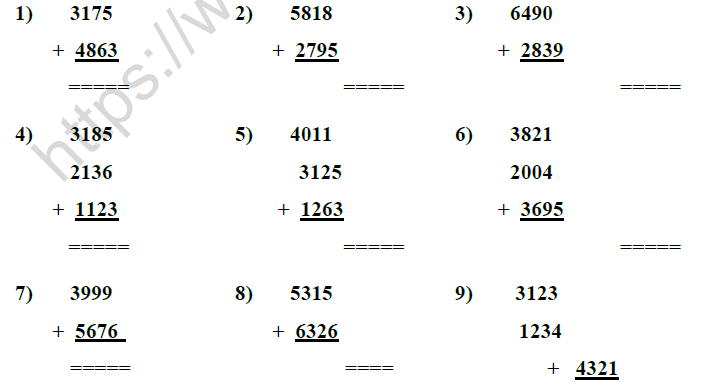# CBSE Class 3 Mathematics Addition and Subtraction MCQs

## MCQ for Class 3 Mathematics Addition and Subtraction

Class 3 Mathematics students should refer to the following multiple-choice questions with answers for Addition and Subtraction in Class 3. These MCQ questions with answers for Class 3 Mathematics will come in exams and help you to score good marks

Q 1) Fill in the blanks :
1. The numbers being added are called ____________________
2. The result of addition of the addends are called the _______________.
3. Zero added to any number is equal to the ______________.
4. Zero subtracted from any number is equal to __________________.
5. While adding if we change the order of the numbers , the result will be the
6. The result of subtraction is called the ________________Q3) Subtract :Q 4)Word Problems based on Addition & Subtraction

1. In three different sectors of a city, the number of people living are 7824, 3625 and 1123 respectively . What is the total number of people living in these sectors ?
2. The population of a village is 4276, 2985 of them are males. Find the population of females.
3. The sum of two numbers is 7345. If one of the numbers is 3456, find the others.
4. Saif earned Rs 9000 in a month and spent Rs 5496. Find his savings ?
5. There are 2972 students in a school in which 1452 are boys. Find the number of the girls?
6. Find the difference between greatest 4 digit number and least 4 digit number.
7. In a village there are 9236 women. The number of men is more than that of the woman by 125. What is the number of men in the village?

I. Fill in the blanks :

1. The numbers which are added are called _______________.

3. Zero added to any number is equal to the ______________.

4. The answer of subtraction is called ______________.

5. Any number - Zero = ______________.

6. Any number - The same number = ______________.

7. 3165 + 0 = ______________.

8. 968 - 0 = ______________.

9. 5818 - 5818 = ______________.

10. 30 + 60 = 60 + ______________.

11. 45 + ( 80 + 90) = 90 + 80 + ______________.

12. 20 + 40 = 60. The addends are ____________ and ____________.

13. 75 + 25 = 100. The sum is ______________.

14. 65 - 25 = 40. Here the difference is ______________.SubtractDo the following

1. In a school there are 5390 boys and 3175 girls. How many students are there in all ?

2. In a garden there are 4120 red rose saplings and 2342 white rose saplings. How many saplings are there in all ?

3. In a school library there 2125 English books, 3280 Science books and 1230 Hindi. How many books are there in all.

4. The population of a village is 5390. Out of them 3125 are males. Find the population of females.

5. In three different sectors of a city the number of people living are 3180, 2496 and 3112 respectively. What is the total number of people living in these sector ?

6. Find the difference between the greatest 4 digit number and the least. 4 digit number.

7. In a school there are 5985 students. On a rainy day 1009 students were absent. How many were present on that day ?

8. The sum of two numbers is 7854. If one of the numbers is 2435, find the other.

## More Study Material

### CBSE Class 3 Mathematics Addition and Subtraction MCQs

We hope students liked the above MCQs for Addition and Subtraction designed as per the latest syllabus for Class 3 Mathematics released by CBSE. Students of Class 3 should download the Multiple Choice Questions and Answers in Pdf format and practice the questions and solutions given in above Class 3 Mathematics MCQs Questions on daily basis. All latest MCQs with answers have been developed for Mathematics by referring to the most important and regularly asked topics which the students should learn and practice to get better score in school tests and examinations. Studiestoday is the best portal for Class 3 students to get all latest study material free of cost.

### MCQs for Mathematics CBSE Class 3 Addition and Subtraction

Expert teachers of studiestoday have referred to NCERT book for Class 3 Mathematics to develop the Mathematics Class 3 MCQs. If you download MCQs with answers for the above chapter daily, you will get higher and better marks in Class 3 test and exams in the current year as you will be able to have stronger understanding of all concepts. Daily Multiple Choice Questions practice of Mathematics and its study material will help students to have stronger understanding of all concepts and also make them expert on all critical topics. You can easily download and save all MCQs for Class 3 Mathematics also from www.studiestoday.com without paying anything in Pdf format. After solving the questions given in the MCQs which have been developed as per latest course books also refer to the NCERT solutions for Class 3 Mathematics designed by our teachers

#### Addition and Subtraction MCQs Mathematics CBSE Class 3

All MCQs given above for Class 3 Mathematics have been made as per the latest syllabus and books issued for the current academic year. The students of Class 3 can refer to the answers which have been also provided by our teachers for all MCQs of Mathematics so that you are able to solve the questions and then compare your answers with the solutions provided by us. We have also provided lot of MCQ questions for Class 3 Mathematics so that you can solve questions relating to all topics given in each chapter. All study material for Class 3 Mathematics students have been given on studiestoday.

#### Addition and Subtraction CBSE Class 3 MCQs Mathematics

Regular MCQs practice helps to gain more practice in solving questions to obtain a more comprehensive understanding of Addition and Subtraction concepts. MCQs play an important role in developing understanding of Addition and Subtraction in CBSE Class 3. Students can download and save or print all the MCQs, printable assignments, practice sheets of the above chapter in Class 3 Mathematics in Pdf format from studiestoday. You can print or read them online on your computer or mobile or any other device. After solving these you should also refer to Class 3 Mathematics MCQ Test for the same chapter

#### CBSE MCQs Mathematics Class 3 Addition and Subtraction

CBSE Class 3 Mathematics best textbooks have been used for writing the problems given in the above MCQs. If you have tests coming up then you should revise all concepts relating to Addition and Subtraction and then take out print of the above MCQs and attempt all problems. We have also provided a lot of other MCQs for Class 3 Mathematics which you can use to further make yourself better in Mathematics

You can download the CBSE MCQs for Class 3 Mathematics Addition and Subtraction for latest session from StudiesToday.com

Yes, you can click on the links above and download topic wise MCQs Questions PDFs for Addition and Subtraction Class 3 for Mathematics

Are the Class 3 Mathematics Addition and Subtraction MCQs available for the latest session

Yes, the MCQs issued by CBSE for Class 3 Mathematics Addition and Subtraction have been made available here for latest academic session